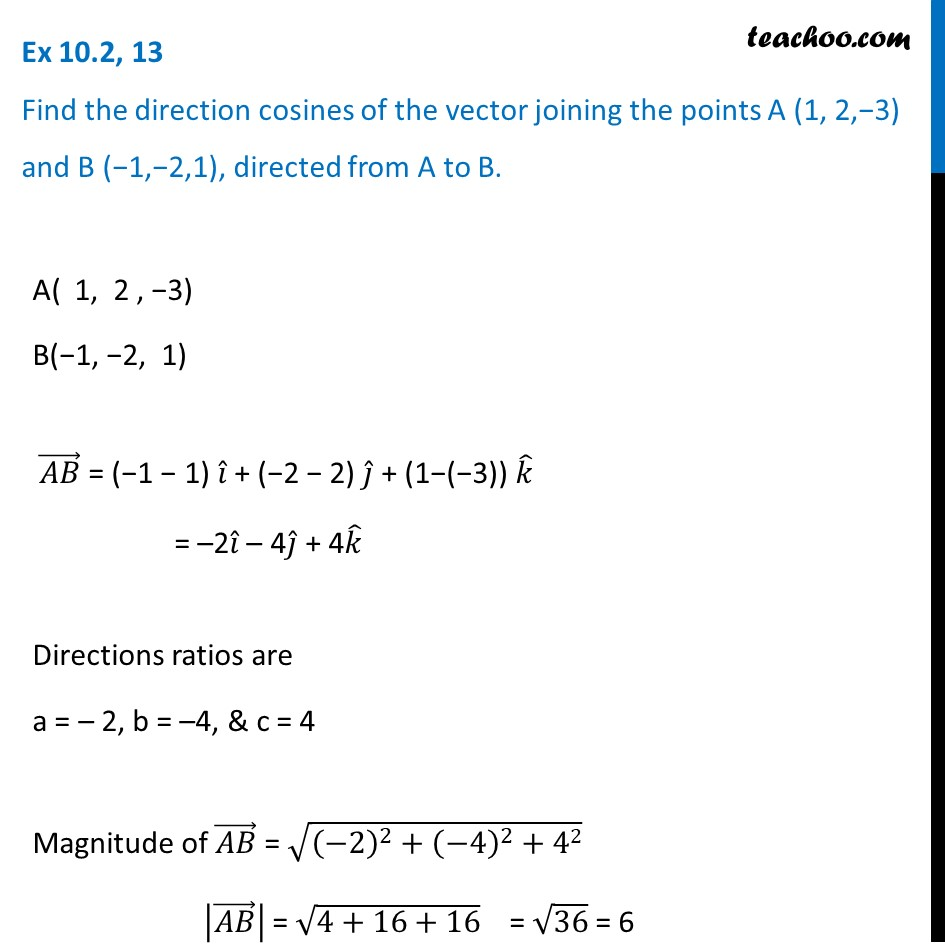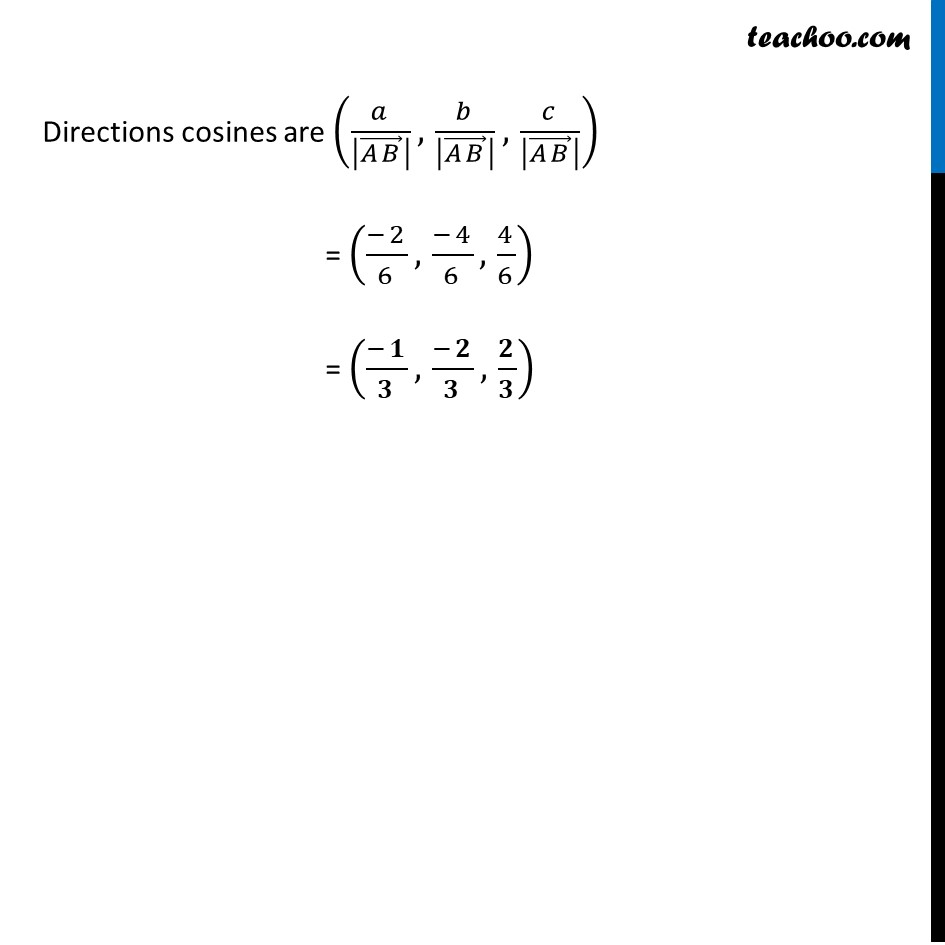Ex 10.2

Chapter 10 Class 12 Vector Algebra
Serial order wiseLearn in your speed, with individual attention - Teachoo Maths 1-on-1 Class

### Transcript

Ex 10.2, 13 Find the direction cosines of the vector joining the points A (1, 2,−3) and B (−1,−2,1), directed from A to B. A( 1, 2 , −3) B(−1, −2, 1) (𝐴𝐵) ⃗ = (−1 − 1) 𝑖 ̂ + (−2 − 2) 𝑗 ̂ + (1−(−3)) 𝑘 ̂ = –2𝑖 ̂ – 4𝑗 ̂ + 4𝑘 ̂ Directions ratios are a = – 2, b = –4, & c = 4 Magnitude of (𝐴𝐵) ⃗ = √((−2)^2+(−4)^2+42) |(𝐴𝐵) ⃗ | = √(4+16+16) = √36 = 6 Directions cosines are (𝑎/|(𝐴𝐵) ⃗ | ,𝑏/|(𝐴𝐵) ⃗ | ,𝑐/|(𝐴𝐵) ⃗ | ) = ((−2)/6,(−4)/6,4/6) = ((−𝟏)/𝟑,(−𝟐)/𝟑,𝟐/𝟑)